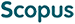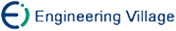陈海鹏, 申铉京, 龙建武. 采用高斯拟合的全局阈值算法阈值优化框架[J]. 计算机研究与发展, 2016, 53(4): 892-903.
 引用本文: 陈海鹏, 申铉京, 龙建武. 采用高斯拟合的全局阈值算法阈值优化框架[J]. 计算机研究与发展, 2016, 53(4): 892-903.Chen Haipeng, Shen Xuanjing, Long Jianwu. Threshold Optimization Framework of Global Thresholding Algorithms Using Gaussian Fitting[J]. Journal of Computer Research and Development, 2016, 53(4): 892-903.
 Citation: Chen Haipeng, Shen Xuanjing, Long Jianwu. Threshold Optimization Framework of Global Thresholding Algorithms Using Gaussian Fitting[J]. Journal of Computer Research and Development, 2016, 53(4): 892-903.## Threshold Optimization Framework of Global Thresholding Algorithms Using Gaussian Fitting

• 摘要: 采用最大类间方差法、最大熵法和最小误差法3种经典全局阈值方法获得的阈值,存在一定偏差.针对该问题,提出了一种采用高斯拟合的全局阈值算法阈值优化框架(TOF).本优化框架先利用全局阈值算法获得初始阈值,将图像粗分为背景和目标2个部分,然后分别计算各部分均值和方差来拟合出2个高斯分布.由于最佳阈值位于2个高斯分布的交点位置,为此本框架采用多次迭代方式来优化阈值,直至最终收敛到最佳阈值.为提高抗噪性能,结合三维直方图重建和降维思想,提出了一种鲁棒的采用高斯拟合的全局阈值算法阈值优化框架(RTOF).实验结果表明,对于以上经典全局算法,采用本优化方法均能收敛到一个最佳阈值,同时本算法还具有鲁棒的抗噪性能和较高的执行效率.

Abstract: There is a certain deviation to obtain the threshold in three classical global thresholding algorithms which are Otsu algorithm, maximum entropy algorithm and minimum error algorithm. To solve this problem, a threshold optimization framework (TOF) of global thresholding algorithms using Gaussian fitting is proposed. Firstly, take advantage of the global threshold method to obtain the initial threshold in the optimization framework and divide the image into two parts of the background and object roughly. And then, Two Gaussian distributions are fitted by calculating the mean and variance of each part. Since the optimal threshold value is in the intersection location of two Gaussian distributions, the presented framework optimizes the thresholds using iterative approach until eventually converging to the optimal threshold position. In order to improve anti-noise performance, combined with the reconstruction of three-dimensional histogram and thinking of reducing the dimensionality, we propose a robust threshold optimization framework (RTOF) of global thresholding algorithms using Gaussian fitting. Finally, extensive experiments are performed and the results show that those thresholds derived from Otsu scheme, maximum entropy scheme and minimum error scheme using the proposed threshold optimization framework can converge to the optimal threshold position. Plus, the presented algorithm has robust anti-noise performance and high-efficiency./下载:  全尺寸图片 幻灯片
• 分享
• 用微信扫码二维码

分享至好友和朋友圈